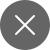# Data Science Interview Questions – Part 2

3+
##### We have covered some essential data science interview questions in our previous article. In this article also, I’ll be covering more such questions.

Let’s start now.

Q.1 What do you understand by logistic regression?

• Logistic regression is easy to use supervised machine learning algorithm. It helps us to predict the categorical dependent variable using a given set of input features.
• Logistic Regression is similar to Linear Regression except for how it works. Linear Regression is useful for solving Regression problems, and Logistic regression helps solve the classification problems.
• In Logistic regression, rather than fitting a regression line, we include an “S” shaped logistic function, which predicts two or more categorical values.

Q.2 Explain dimensionality reduction?

Dimensionality reduction involves converting a dataset with many dimensions (fields) to a dataset having a low number of sizes. This is done by dropping less essential features from the dataset. However, this is not done arbitrarily. In this process, the dimensions or fields are dropped only after ensuring that the remaining information will still be enough to describe similar details briefly.

The gradient shows the direction and magnitude determined during the training of a neural network. These parameters are used to update the network weights in the right order and by the right measure.

Exploding gradients is a problem where large error gradients expand and lead to a substantial change in neural network model weights during training. At an absolute, the weights’ values can grow so large as to overflow and end in NaN values.

This affects your model, and it becomes unable to learn from the training data.

Q.4  How can we avoid overfitting in the machine learning model?

Overfitting occurs in a model that learns from a minimal amount of data and neglects the bigger picture. The three essential methods to avoid overfitting are:

• Make the model simple, considering essential features, removing irrelevant information (noise) in the training data.
• We can use cross-validation techniques, like K-folds cross-validation.
• We can also use various regularization techniques, such as LASSO, that penalize our model parameters if they’re willing to cause overfitting.

Q.5  What do you mean by Normal Distribution?

Data is typically distributed differently with a bias either to the left or right or randomly distributed.

However, there are cases in that; data may be distributed around a central value without any bias in either direction. It reaches normal distribution in the shape of a bell-shaped curve.

Here, we can see that the variables are distributed in the form of a bell-shaped curve. The normal distribution measures the mean value, half of the data remains to the left of the curve, and the remaining half lies right. The normal distribution is also known as Gaussian distribution, to determine probability distribution. The two important parameters of the normal distribution are mean(µ) and standard deviation(σ).

Q.6 Explain correlation and covariance in statistics?

Covariance is a statistical approach, defines the systematic relationship between a pair of random variables. Here, a change in one variable leads to an equivalent change in another variable.

Covariance takes any value between -∞ to +∞. The negative value indicates a negative relationship, essential whereas a positive value shows a positive association. Further, it represents the linear relationship among variables. When the value is zero, it shows that variables are not related.

Correlation is defined as a metric in statistics. It indicates the degree to which two or more random variables change in the cycle. While studying two variables, if it has been seen that the change in one variable is reciprocated by an equivalent shift to another variable, in one or the other manner, then we can say that the variables are correlated.

Q.7 Explain the Naive Bayes algorithm. Also, why it is known as Naive?

Naive Bayes is a machine learning algorithm used for solving classification problems. It works on the concept of the Bayes Theorem. It is easy to understand but powerful ML algorithms. It is useful for various applications. It is a probabilistic classifier,i.e., it uses the probability of an object for prediction.

It is called naive because it assumes that the model’s features are independent of each other. It means changing the value of one feature will not influence or change any of the other features used in the algorithm.

Q.8 How Regression and classification techniques are different from each other?

Both Regression and classification machine learning techniques are parts of Supervised machine learning algorithms. In a Supervised machine learning algorithm, we train our model using a labeled data set.  We have to explicitly provide the correct labels during training and the model tries to learn the pattern from given data. If the target labels have discrete values, then it is a classification problem, e.g. 0, 1, etc., but if the target label has continuous values, then it is a regression problem, e.g. 34.56, 18.333, etc.

We have covered the most important questions in this article. Stay connected for more such amazing articles.3+

### 1 Response

1. March 10, 2021

[…] Also, check Data Science Interview Questions – Part 1 and Data Science Interview Questions – Part 2 […]

0